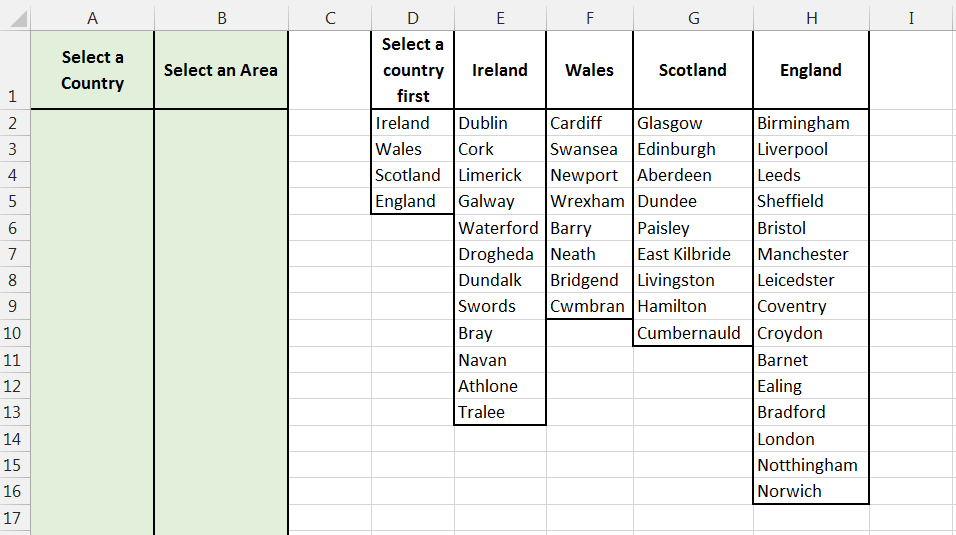# Data Validation List Based On CriteriaIn earlier posts, we covered data validation list, from basic to more complex rules.  In this post, we will create a rule for a Data Validation List that will be dependent on another value.

If you want to read back over earlier posts, you can click on the links below.

What do I mean by this?

If we use an example where I have 2 columns:

1. Column A will have a simple Data Validation List rule that will allow the user to select a country from column D
2. Column B will be the focus of this post.  The rule for the Data Validation List will be dependent on what country is selected in column A.If the country in column A is Ireland, then the list in column B will only list the locations from column E (Ireland).

You will only see the list options that you need to see, you won’t have to scroll through all locations for all countries (as you would have to if a simple rule was set up based on a list of locations).

An Intermediate to Advanced level of Excel knowledge may be needed here if you want to recreate this rule yourself.  For the sake of demonstration I have put the sources to all my Data Validation List Rules on the same sheet as the cells that I will be applying the rules too.  Normally i would not do this, I would have them on a different sheet.

How do we do this?

The source for our list will be based on an IF statement.For cell B2, we are essentially going to say:

• If A2 is Ireland, then the source will be from column E
• Or, If A2 is Wales, then the source will be from column F
• Or, If A2 is Scotland, then the source will be from column G
• Or, If A2 is England, then the source will be from column H
• Or Else (i.e. none of the above countries are selected in A2) the only option available is “Select a country first”

The full IF function will be:  =IF(A2=”Ireland”,\$E\$2:\$E\$13,IF(A2=”Wales”,\$F\$2:\$F\$9,IF(A2=”Scotland”,\$G\$2:\$G\$10,IF(A2=”England”,\$H\$2:\$H\$16,\$D\$1))))

To understand and read the function note the commas, the use of \$ and brackets (each opening bracket has a corresponding closing bracket).

If building your own rule like this, I suggest building it piece by piece.  In my case above, I created the first IF function, if A2 is “Ireland” then E2:E13 or else D1.

When I was happy with that, I amended the value_if_false to be another IF function, this time checking if the country is Wales and so on.

The first time I was asked for a Data Validation List rule like this it was for a situation where the drop-down list had to be changed based on a numeric value.  If the number was below the target then the list should give main reasons why the target wasn’t reached, or else it was to be blank because a comment wasn’t required.

Could you replicate a rule like this?